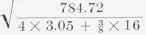Fig. 91. Detail of Vertical Hoist Door.Fig. 92. Detail of Rolling Door of Corrugated Steel. Courtesy of Kinnear Mfg. Co., Columbus, Ohio.Fig. 93. Cross-Sections of Columns.

In order to prevent eccentric stresses due to the reaction of the runway girder, an extra column to carry the crane girder is placed alongside the roof column (see Figs. 100 and 117, pp. 75 and 88). This is much used by A. F. Robinson, Bridge Engineer of the Atchison, Topeka & Santa Fe Railroad System, who claims it to be a very economical and efficient detail. One advantage of this is, that, if at any time it is desirable to use a heavier crane, this column can be removed and a stronger one put in its place, without in any way affecting the remainder of the building.Fig. 94. Illustrating the Two Radii of Gyration.Fig. 95. Runway-Girder Eccentricity.

In order to illustrate the design of a column, let it be required to design a column with detail as shown in Fig. 95, the height being 20 feet, the distance of the runway girder from the face of the column 8 inches, the direct stress 15 600 pounds, and the bending moment due to the wind 924 000 pound-inches. The reaction of the runway girder is 20 000 pounds. The stress due to the bending moment caused by the wind and the eccentricity of the runway girder must be worked out by formula 8, "Strength of Materials," p. 86; and to this must be added the direct stress caused by the weight of the roof and the crane-girder reaction.

Since, according to Article 13, the unit-stress must be reduced one-half in determining the section to withstand stresses due to crane loads, the moment due to the crane loads and also its direct action must be multiplied by 2 in order that the same formula for the unit-stress may be used throughout in the design of the column.Fig. 96. Runway-Girder and Roof-Truss Eccentricity.Fig. 97. Illustrating Problem on Page 73.

Let four 5 by 3½ by ⅜-inch angles with a 16 by ⅜-inch web plate be assumed, and placed as shown in Fig. 97. These angles have an area of 3.05 square inches each; and a moment of inertia parallel to the long leg, of 3 18. Then (see "Strength of Materials," pp. 48-53), the moment due to crane reaction is 20 000 X (8.125 + 8) = 322 500 pound-inches. Accordingly, in using this in the formula, it will be 2 X 322 500 = 645 000 pound-inches; and this, added to the 924 000 pound-inches due to the wind, will make a total inches.Fig. 98. Detail of Column.

bending moment of 1,569,000 pound-

I a-a = 4 X3.18 + 4 X 3.05X 7.2652 + 3/8 X16 /12 = 784.72, ra-a==6.56

The allowable unit-stress is:

P = 24 000- 110 X 20 X 12/ 6.56 = 19 075 pounds per square inch.Fig. 99. Detail of Column.Fig. 100. Detail of Column.

The actual unit-stress (see "Strength of Materials," p. 86), is:

S = 15600 + 2 X20000 / 4X3.05 + 3/8 X16 + 1.569000 X16.25/2 / 784.72- (15 600 + 2 X 20 000) (20 X 12)2 / 10 X 28 000 000

= 3 024 + 16 420

= 19 444 pounds per square inch.

Since this is slightly less than the allowable stress, 19 975 pounds per square inch, this section is the correct one.

Details of columns are shown in Figs. 98, 99, and 100. In case the column is considered fixed at its base, the base of the column is usually made as shown in Fig. 99. Long bolts deeply imbedded in the masonry are run up through the holes a; a heavy washer is placed over the bolt, and the nut screwed down tightly. Each bolt must be designed to withstand a stress of H2 X n ÷ 2d (see Figs. 43 and 99). 24. Knee=Braces. The determination of the stresses in knee-bracing has been made in Article 9. Knee-braces consist of two angles placed back to back, and are joined to the column and roof truss as shown in Fig. 101 and in the figures showing cranes. They must be designed to withstand the greatest compressive stress; and must also be examined to see if they are safe in tension, since they are under either tensile or compressive stresses according to the direction in which the wind blows.

The knee-brace for the truss-bent of Article 9 will now be designed. The maximum compressive stress is 21 440 pounds. The radius of gyration must be at least 131 ÷ 120 = 1.09. Two angles 3½ by 3 by 5/16-inch, placed back to back with their longer legs ¼ inch apart, will be assumed, since they are the smallest size to be used with r = 1.09 or greater. The radius of gyration about an axis perpendicular to the longer legs is 1.10; and the allowable unitstress is P 24 000 - 110 X 131/ 110 = 10 900 pounds per square

21 440/10 900 inch, the length being 131 inches. The required area is

= 1.97 square inches. Since this is less than the given area, and since the angles are the smallest allowed, these angles are sufficient. The maximum tensile stress is 8 640 pounds, and the required net area is 8 640 ÷15 000 = 0.58 square inch. The net area given by the angles is 3.80 - 0.55 = 3.31 square inches, two ¾-inch rivet-holes being taken out. This shows the angles to be amply safe in tension, and they will therefore be used for the section of the knee-braces.